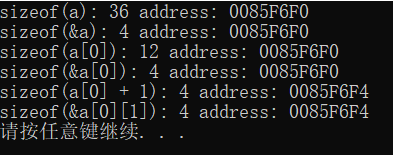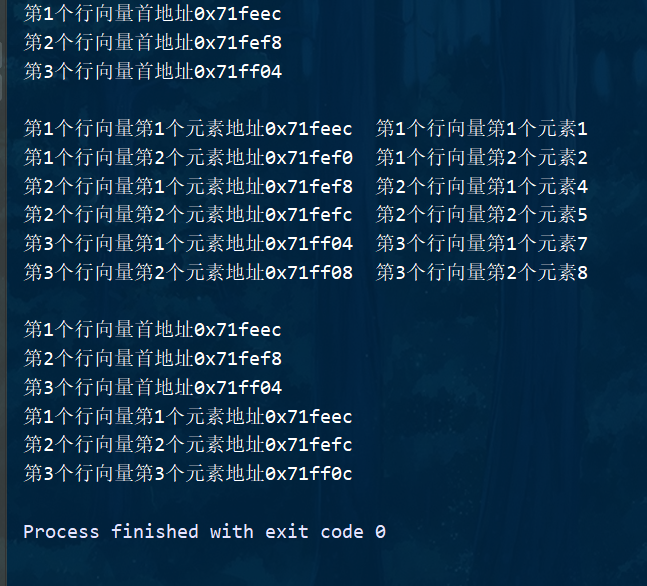2021-05-05 13:44

# 【二维数组问题】二维数组名含义问题

a代表首行地址，一般用a地址表示；

&a代表整个数组的地址，一般用a地址表示；

a[i]代表第i行起始元素的地址；

&a[i]代表第i行的地址，一般用a[i]地址表示；

``````#include <iostream>
using namespace std;
int main()
{
int a = {
{1, 2, 3},
{4, 5, 6},
{7, 8, 9}
};
cout << "sizeof(a): " << sizeof(a) << " address: " << a << endl;
cout << "sizeof(&a): " << sizeof(&a) << " address: " << &a << endl;
cout << "sizeof(a): " << sizeof(a) << " address: " << a << endl;
cout << "sizeof(&a): " << sizeof(&a) << " address: " << &a << endl;
cout << "sizeof(a + 1): " << sizeof(a + 1) << " address: " << a + 1 << endl;
cout << "sizeof(&a): " << sizeof(&a) << " address: " << &a << endl;
system("pause");
return 0;
}``````• 点赞
• 写回答
• 关注问题
• 收藏
• 复制链接分享
• 邀请回答

#### 4条回答

• 先说第一个，sizeof(a)不是指整个数组地址，而是指这个二维数组所占内存的大小，因为你的是int整型数组，每个整型占4个字节，一共9个元素，所以占36个字节；
再说sizeof(&a)，这个求解的是数组a所在的地址占的内存大小，这里你可以理解成指针，因为指针的本质就是地址，你可以去百度查，规定指针类型的内存大小为你使用的计算机的位数所占的字节，这里我猜测你的电脑应该是32位即4字节的，所以显示为4；我的电脑使用64位，所以显示为8；
再来说a、&a、a、&a的地址关系，数组在C语言中是顺序排列的，所以最开始的元素不就是a吗，同时这个元素所占的地址不也是整个数组的起始地址a吗，同时c语言里面数组名是可以当地址使用的，&a的地址即为a的地址。a是二维数组的第一个一位数组，那么它即是一个数组名，也可以当做地址使用。
这些地址都是对的，你用哪个都可以，具体使用哪个无非是看你题目的要求。你的a+1和&a都是指元素a所在的地址，所以地址显示相同
这里特别重要的知识点就是，当有&或者*符号时，都是当做指针来计算，指针的大小与你的类型、长度无关，只与你的操作系统所占位数有关

点赞 1 评论 复制链接分享
• 也许对你有帮助：https://blog.csdn.net/it_xiangqiang/category_10581430.html

点赞 1 评论 复制链接分享
• a数组名，代表整个数组，它指向的是整个数组占用的地址空间

&a代表整个数组的的第一个元素的地址，也就是起始地址

a[i]代表第i行数组元素，它指向的是第i行占用的地址空间

&a[i]代表第i行的第一个元素的地址，也就是第i行的起始地址

a[i][j]代表的第i行第j列的元素

&a[i][j]代表的第i行第j列的地址

希望对你有用

点赞 1 评论 复制链接分享
• ``````#include <iostream>
using namespace std;
int main()
{
int a = {
{1, 2, 3},
{4, 5, 6},
{7, 8, 9}
};
cout << "第1个行向量首地址" << *(a+0) <<endl;
cout << "第2个行向量首地址" << *(a+1)<<endl;
cout << "第3个行向量首地址" << *(a+2) <<endl;

cout << endl;
cout << "第1个行向量第1个元素地址" << *(a+0) +0 <<"  第1个行向量第1个元素" << *(*(a+0)+0)<<endl;
cout << "第1个行向量第2个元素地址" << *(a+0) +1 <<"  第1个行向量第2个元素" << *(*(a+0)+1)<<endl;
cout << "第2个行向量第1个元素地址" << *(a+1) +0 <<"  第2个行向量第1个元素" << *(*(a+1)+0)<<endl;
cout << "第2个行向量第2个元素地址" << *(a+1) +1 <<"  第2个行向量第2个元素" << *(*(a+1)+1)<<endl;
cout << "第3个行向量第1个元素地址" << *(a+2) +0 <<"  第3个行向量第1个元素" << *(*(a+2)+0)<<endl;
cout << "第3个行向量第2个元素地址" << *(a+2) +1 <<"  第3个行向量第2个元素" << *(*(a+2)+1)<<endl;

cout << endl;
cout << "第1个行向量首地址" << &a << endl;
cout << "第2个行向量首地址" << &a << endl;
cout << "第3个行向量首地址" << &a << endl;
cout << "第1个行向量第1个元素地址" << &a << endl;
cout << "第2个行向量第2个元素地址" << &a << endl;
cout << "第3个行向量第3个元素地址" << &a << endl;

return 0;
}``````点赞 评论 复制链接分享